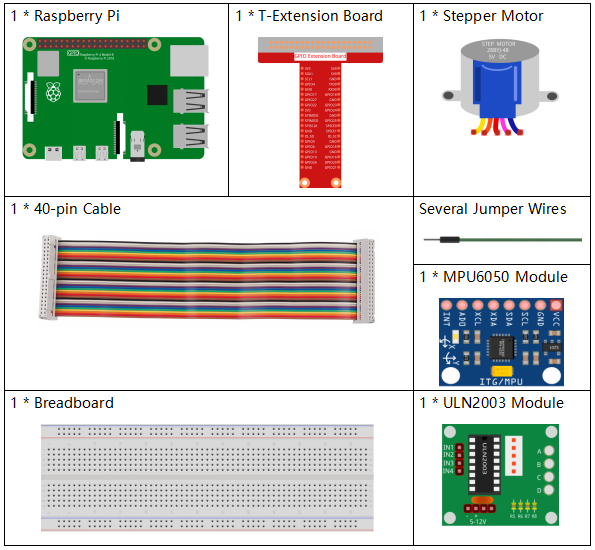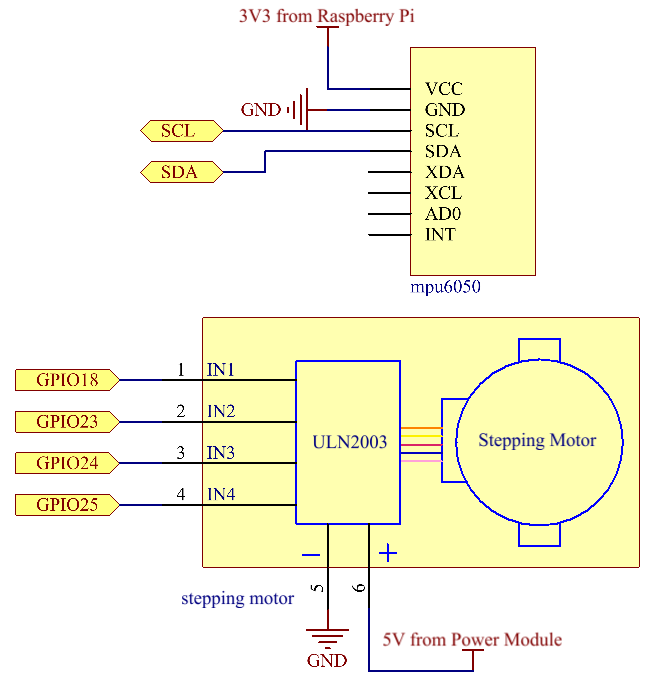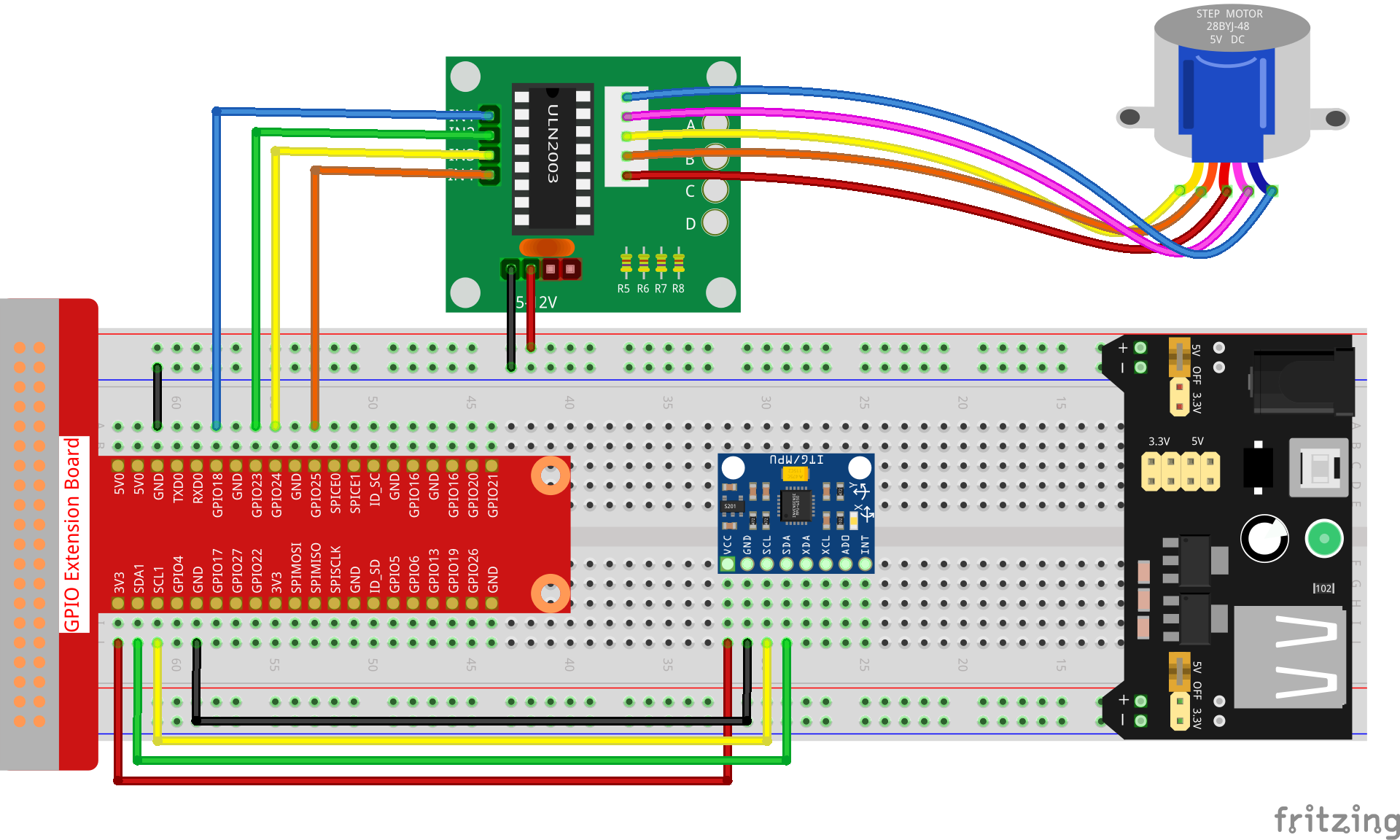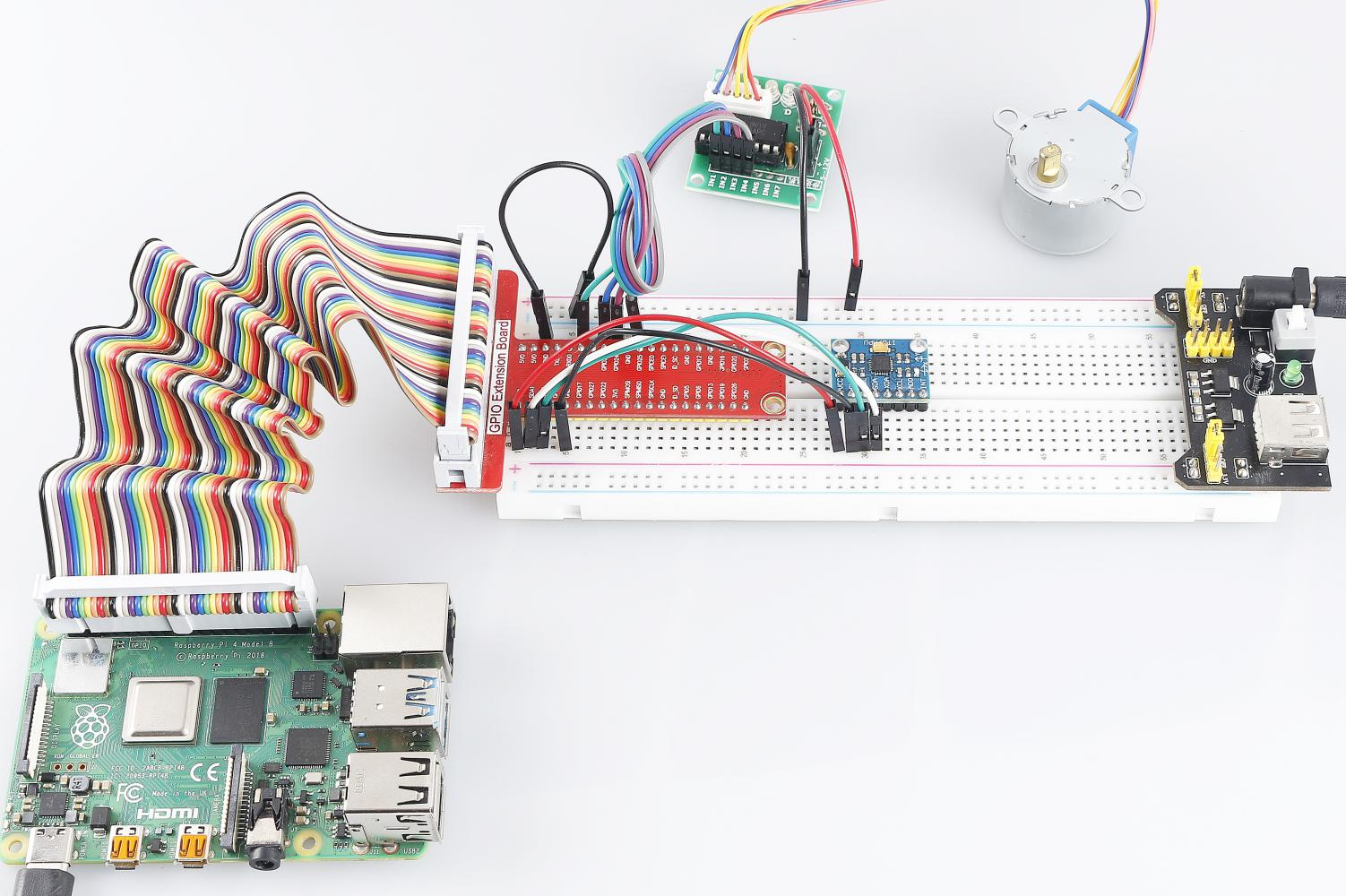# 3.1.6 Motion Control¶

## Introduction¶

In this lesson, we will make a simple motion sensing and controlling device. The MPU6050 is used as a sensor and the stepper motor as a controlled device. With the MPU6050 mounted on the glove, you can control the stepper motor by rotating your wrist.

## Components¶## Schematic Diagram¶

 T-Board Name physical wiringPi BCM GPIO18 Pin 12 1 18 GPIO23 Pin 16 4 23 GPIO24 Pin 18 5 24 GPIO25 Pin 22 6 25 SDA1 Pin 3 SCL1 Pin 5## Experimental Procedures¶

Step 1: Build the circuit.### For C Language Users¶

Step 2: Go to the folder of the code.

```cd ~/davinci-kit-for-raspberry-pi/c/3.1.6/
```

Step 3: Compile the code.

```gcc 3.1.6_MotionControl.c -lwiringPi -lm
```

Step 4: Run the executable file.

```sudo ./a.out
```

As the code runs, if the tilt angle of mpu6050 on the Y-axis is larger than 45 ℃, the stepper motor rotates anticlockwise; if less than -45 ℃, the stepper motor rotates clockwise.

Note

If it does not work after running, or there is an error prompt: "wiringPi.h: No such file or directory", please refer to C code is not working?.

Code Explanation

```double mpu6050(){
acclX_scaled = acclX / 16384.0;
acclY_scaled = acclY / 16384.0;
acclZ_scaled = acclZ / 16384.0;
double angle=get_y_rotation(acclX_scaled, acclY_scaled, acclZ_scaled);
return angle;
}
```

mpu6050 gets the tilt angle in the direction of the Y-axis.

```void rotary(char direction){
if(direction == 'c'){
for(int j=0;j<4;j++){
for(int i=0;i<4;i++)
{digitalWrite(motorPin[i],0x99>>j & (0x08>>i));}
delayMicroseconds(stepSpeed);
}
}
else if(direction =='a'){
for(int j=0;j<4;j++){
for(int i=0;i<4;i++)
{digitalWrite(motorPin[i],0x99<<j & (0x80>>i));}
delayMicroseconds(stepSpeed);
}
}
}
```

If the received direction key is 'c', the stepper motor rotates clockwise; if the key is 'a', the motor rotates anticlockwise. Refer to 1.3.3 Stepper Motor for more details about the calculation of the rotating direction of the stepper motor.

```int main()
{
setup();
double angle;
while(1) {
angle = mpu6050();
if (angle >=45){rotary('a');}
else if (angle<=-45){rotary('c');}
}
return 0;
}
```

The tilt angle in the direction of the Y-axis is read from mpu6050, and if it’s larger than 45 ℃, the stepper motor rotates anticlockwise; if less than -45 ℃, the stepper motor rotates clockwise.

### For Python Language Users¶

Step 2: Go to the folder of the code.

```cd ~/davinci-kit-for-raspberry-pi/python/
```

Step 3: Run the executable file.

```sudo python3 3.1.6_MotionControl.py
```

As the code runs, if the tilt angle of mpu6050 on the Y-axis is larger than 45 ℃, the stepper motor rotates anticlockwise; if less than -45 ℃, the stepper motor rotates clockwise.

Code

Note

You can Modify/Reset/Copy/Run/Stop the code below. But before that, you need to go to source code path like `davinci-kit-for-raspberry-pi/python`.

```import RPi.GPIO as GPIO
import smbus
import math
import time

# Power management registers
power_mgmt_1 = 0x6b
power_mgmt_2 = 0x6c

bus = smbus.SMBus(1)

#Stepper motor pins
motorPin = (18,23,24,25)
rolePerMinute =15
stepsPerRevolution = 2048
stepSpeed = (60/rolePerMinute)/stepsPerRevolution

#mpu6050

val = (high << 8) + low
return val

if (val >= 0x8000):
return -((65535 - val) + 1)
else:
return val

def dist(a,b):
return math.sqrt((a*a)+(b*b))

def get_y_rotation(x,y,z):

def get_x_rotation(x,y,z):

def mpu6050():
accel_xout_scaled = accel_xout / 16384.0
accel_yout_scaled = accel_yout / 16384.0
accel_zout_scaled = accel_zout / 16384.0
angle=get_y_rotation(accel_xout_scaled, accel_yout_scaled, accel_zout_scaled)
return angle

#Stepper Motor
def rotary(direction):
if(direction == 'c'):
for j in range(4):
for i in range(4):
GPIO.output(motorPin[i],0x99>>j & (0x08>>i))
time.sleep(stepSpeed)

elif(direction == 'a'):
for j in range(4):
for i in range(4):
GPIO.output(motorPin[i],0x99<<j & (0x80>>i))
time.sleep(stepSpeed)

def setup():
GPIO.setwarnings(False)
GPIO.setmode(GPIO.BCM)
for i in motorPin:
GPIO.setup(i, GPIO.OUT)

def loop():
while True:
angle=mpu6050()
if angle >=45 :
rotary('a')
elif angle <=-45:
rotary('c')

def destroy():
GPIO.cleanup()

if __name__ == '__main__':
setup()
try:
loop()
except KeyboardInterrupt:
destroy()
```

Code Explanation

```def mpu6050():
accel_xout_scaled = accel_xout / 16384.0
accel_yout_scaled = accel_yout / 16384.0
accel_zout_scaled = accel_zout / 16384.0
angle=get_y_rotation(accel_xout_scaled, accel_yout_scaled, accel_zout_scaled)
return angle
```

mpu6050 gets the tilt angle in the direction of the Y-axis.

```def rotary(direction):
if(direction == 'c'):
for j in range(4):
for i in range(4):
GPIO.output(motorPin[i],0x99>>j & (0x08>>i))
time.sleep(stepSpeed)

elif(direction == 'a'):
for j in range(4):
for i in range(4):
GPIO.output(motorPin[i],0x99<<j & (0x80>>i))
time.sleep(stepSpeed)
```

If the received direction key is 'c', the stepper motor rotates clockwise; if the key is 'a', the motor rotates anticlockwise. Refer to 1.3.3 Stepper Motor for more details about the calculation of the rotating direction of the stepper motor.

```def loop():
while True:
angle=mpu6050()
if angle >=45 :
rotary('a')
elif angle <=-45:
rotary('c')
```

The tilt angle in the direction of the Y-axis is read from mpu6050, and if it’s larger than 45 ℃, rotary() is called to let the stepper motor rotate anticlockwise; if less than -45 ℃, the stepper motor rotates clockwise.

## Phenomenon Picture¶